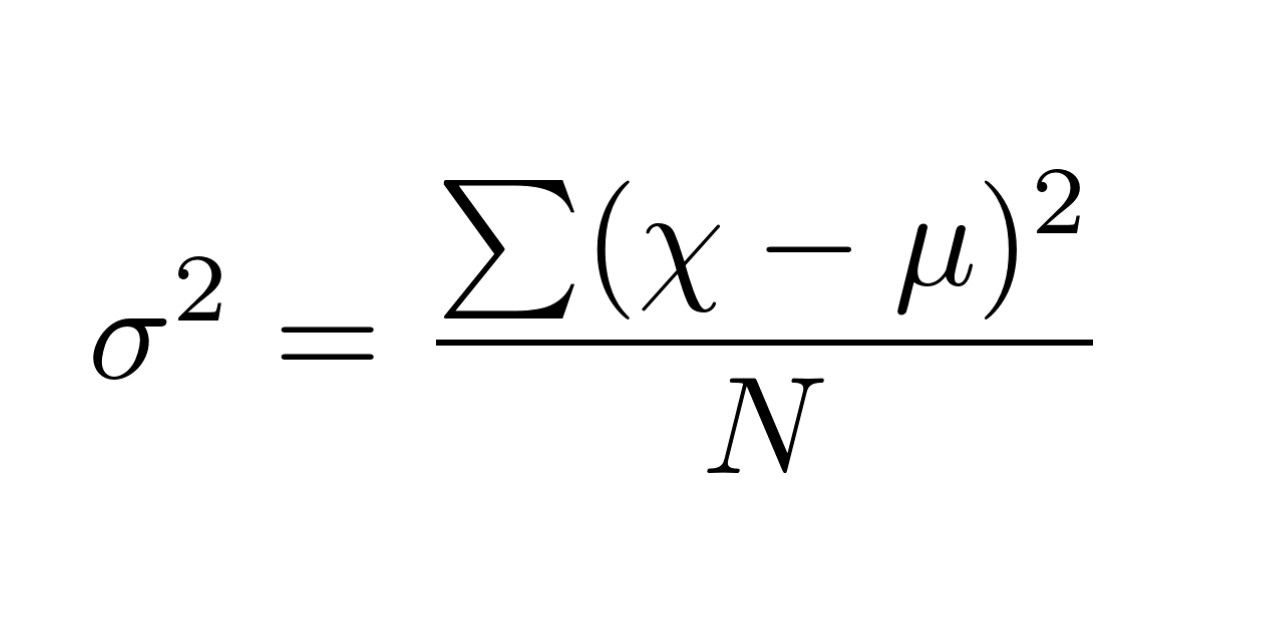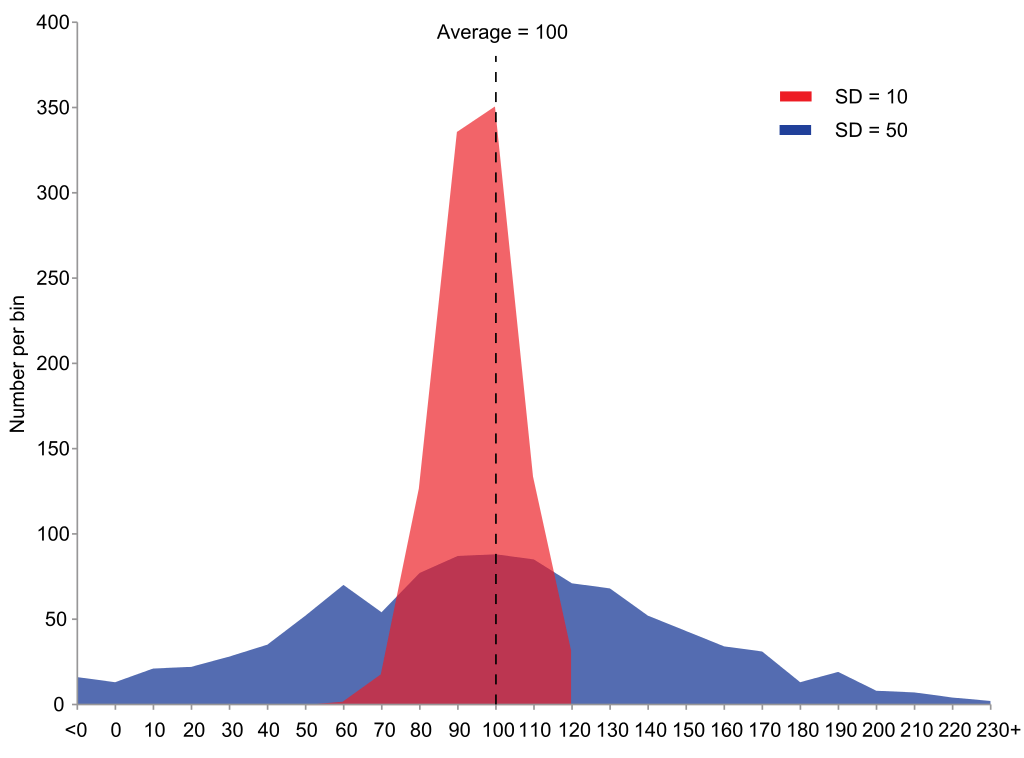# Variance

## What is Variance?

Variance is the expected value of the squared deviation of a random variable from its mean. In short, it is the measurement of the distance of a set of random numbers from their collective average value. Variance is used in statistics as a way of better understanding a data set's distribution.

## How does Variance work?

Variance is calculated by finding the square of the standard deviation of a variable, and the covariance of the variable with itself, as represented by the function:In the formula above, u represents the mean of the data points, x is the value of an individual data point, and N is the total number of data points. It is important to note that because the formula works with the square of the deviations, variance is always going to be a positive number, or zero. If the variance is zero, then all entries likely have the same value. Similarly, a large variance infers that the numbers in the set are far from the mean and each other. Additionally, the variance formula can be amended such that if the data set values are scaled by a constant, for example, then the variance is scaled by the square of that constant.By JRBrown - Own work, Public Domain, https://commons.wikimedia.org/w/index.php?curid=10777712

Variance is used often in statistics as a way of better understanding a data set's distribution. A disadvantage of variance is that it places emphasis on outlying values (that are far from the mean), and the square of these numbers can skew conclusions about the data. However, due to the non-negative principle of variance, one will always be able to interpret variability, as all deviations from the mean are calculated equally, regardless of direction.

## Applications of Variance

Variance is an extremely useful arithmetic tool for statisticians and data scientists alike. As a function for understanding distribution, variance is applicable in disciplines from finance, to machine learning.

### Variance and Finance

Investors use variance calculations in asset allocation. By calculating the variance of asset returns, investors and financial managers can better develop optimal portfolios by maximizing the return-volatility trade-off. Often however, risk is understood by the standard variation, rather than variance, as it is easier to interpret and understand.

### Variance and Machine Learning

As a statistical tool, data scientists often use variance to better understand the distribution of a data set. Machine learning uses variance calculations to make generalizations about a data set, aiding in a neural network's understanding of data distribution. Variance is often used in conjunction with probability distributions.